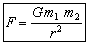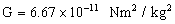# Physics Assignment Help With Gravitation

## 6.1 Newton's law of Gravitation

According to Newton's laws of gravitation the force of attraction between two point masses is directly proportional to product of their masses and inversely proportional to square of distance between them.where G is a universal gravitational constant. The value of G.Regarding gravitational force it is worth noting that:

(1) It is always attractive [while electric and magnetic force can be attractive or repulsive.]

(2) It is independent of the medium between the particles (while electric and magnetic force depend on the nature of the medium between the particles).

(3) It holds good over a wide range of distances. It is found true from interplanetary distances to inter-atomic distances.

(4) It is an action-reaction pair, i.e., the force with which one body (say earth) attracts the second body (say moon) is equal to the force with which moon attracts the earth. (However, accelerations, will not be equal).

(5) It is a two-body interaction, * i.e., gravitational force between two particles is independent of the presence or absence of other particles; so the principle of superposition is valid, i.e., force on a particle due to number of particles is the resultant of forces due to individual particles, i.e.

### Email Based Assignment Help in Gravitation

We are the leading online Assignment Help provider. Find answers to all of your doubts regarding the Gravitation. Assignmenthelp.net provides homework, Assignment Help to the school, college or university level students. Our expert online tutors are available to help you in Gravitation. Our service is focused on: time delivery, superior quality, creativity and originality.